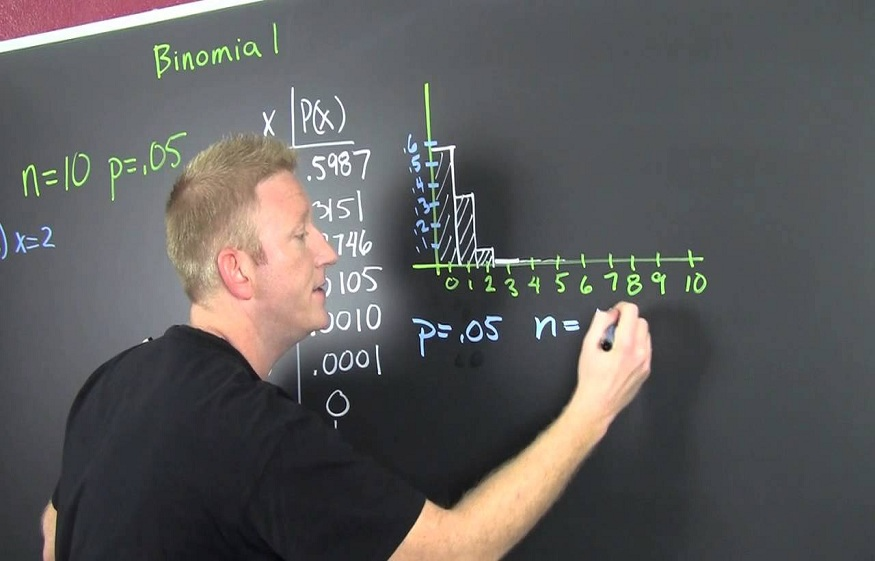# Real-life Applications of Probability Distribution179 Views

Probability distributions are defined as the mathematical functions illustrating probabilities of things occurring. Probability has thousands of uses and applications that we may or may not know in our daily existence. In the same way, there exist numerous applications for probability distributions. As we know, multiple functions take place in the world around us every day. All these can be described by a handful of distributions that have been well-researched and studied. We can observe many practical uses of probability distributions in our real-life events.

We can define many real-life applications of different types of probability distributions. For example, when a new drug is introduced to cure a particular disease, it may either cure the disease (i.e., it is successful) or doesn’t cure it (i.e., it is a failure). Consider another example, suppose you purchase a lottery ticket, then there are two possibilities you will win the money or don’t win. So, basically, anything you can think of that can only be a success or a failure, and a binomial distribution can represent this. Similarly, we can discuss multiple real-life examples where we apply probability distributions.

Another empirical distribution is the Poisson distribution. Like the binomial, it is also probability distribution, indicating a finite set of probable outcomes. In the case of binomial, there were just two outcomes: yes or no. When it comes to the Poisson distribution, there might be more, i.e., more than two, though they can only be whole numbers: 0, 1, 2, 3, etc. Thus, we use the Poisson distribution to define events that happen at some rate over a period of time or space. Poisson distribution is also used for modelling the random arrival, such as the arrival of individuals at the queues or calls at the help desks.

A distribution closely connected to Poisson is the exponential distribution. If the number of events happening in some time follows the Poisson technique, then the time between those events is represented by the exponential distribution. The normal distribution is the most important probability distribution as it fits many natural phenomena. Some examples include the height of pupils, shoe sizes, blood pressure, income distribution of an economy, IQ score of a particular population, etc. This type of probability distribution is also called a Gaussian distribution. This distribution is a bell-shaped curve encompassing two basic terms: mean and standard deviation.

Till now, we have discussed various types of probability distributions, but all these are divided into two major categories, discrete probability distribution and continuous probability distribution. All these can be defined for many real-life scenarios. Let us know some real-life examples of the continuous probability distribution. The day’s temperature can be considered one of the real-life examples of the continuous probability distribution. Thus, we have a large number of applications for the probability distribution in our daily existence. Some include mathematical, some will be scientific, and some will be included in the general functions. All these can be estimated using certain specific formulas and factors.